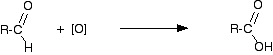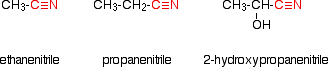Need help? Email help@shout.education

# Making Carboxylic Acids

This page looks at ways of making carboxylic acids in the lab by the oxidation of primary alcohols or aldehydes, and by the hydrolysis of nitriles.

Note: If you are interested in the preparation of benzoic acid (benzenecarboxylic acid) you will find it described in the section on arenes (aromatic hydrocarbons like benzene and methylbenzene). Benzoic acid is normally made by oxidising hydrocarbon side chains attached to a benzene ring. This is described towards the bottom of the page you will get to by following this link.

## Making Carboxylic Acids by Oxidising Primary Alcohols or Aldehydes

### Chemistry of the Reactions

Primary alcohols and aldehydes are normally oxidised to carboxylic acids using potassium dichromate(VI) solution in the presence of dilute sulfuric acid. During the reaction, the potassium dichromate(VI) solution turns from orange to green.

The potassium dichromate(VI) can just as well be replaced with sodium dichromate(VI). Because what matters is the dichromate(VI) ion, all the equations and colour changes would be identical.

Primary alcohols are oxidised to carboxylic acids in two stages – first to an aldehyde and then to the acid. We often use simplified versions of these equations using "[O]" to represent oxygen from the oxidising agent.

##### The formation of the aldehyde is shown by the simplified equation:"R" is a hydrogen atom or a hydrocarbon group such as an alkyl group.

Note: Although "R" can in principle be a hydrogen atom, in practice if it is a hydrogen, the oxidation eventually goes all the way to carbon dioxide and water rather than stopping at methanoic acid. Unlike most other carboxylic acids, methanoic acid is very easily oxidised.

The aldehyde is then oxidised further to give the carboxylic acid:If you start with an aldehyde, you are obviously just doing this second stage.

Starting from the primary alcohol, you could combine these into one single equation to give:

RCH_2OH + 2[O] \longrightarrow RCOOH + H_2O

For example, if you were converting ethanol into ethanoic acid, the simplified equation would be:

CH_3CH_2OH + 2[O] \longrightarrow CH_3COOH + H_2O

It is possible that you might want to write proper equations for these reactions rather than these simplified ones. You can work these out from electron-half-equations. How you do this is described in detail elsewhere on the site.

##### The complete equation for the conversion of a primary alcohol to a carboxylic acid is:
3RCH_2OH + {2\text{Cr}_2O_7}^{2-} + 16H^+ \longrightarrow 3RCOOH + 4\text{Cr}^{3+} + 11H_2O
##### or if you were starting from an aldehyde is:
3RCHO + {\text{Cr}_2O_7}^{2-} + 8H^+ \longrightarrow 3RCOOH + 2\text{Cr}^{3+} + 4H_2O

Note: The equation for the conversion of ethanol to ethanoic acid is worked out in detail on the page about electron-half-equations mentioned above. It isn't difficult to work out the aldehyde one using the same principles – it would be a useful exercise to check that you understand what to do.

### Doing the Reactions

It would actually be quite uncommon to make an acid starting from an aldehyde, but very common to start from a primary alcohol. The conversion of ethanol into ethanoic acid would be a typical example.

The alcohol is heated under reflux with an excess of a mixture of potassium dichromate(VI) solution and dilute sulfuric acid.

Heating under reflux (heating in a flask with a condenser placed vertically in it) prevents any aldehyde formed escaping before it has time to be oxidised to the carboxylic acid.

Using an excess of oxidising agent is to be sure that there is enough oxidising agent present for the oxidation to go all the way to the carboxylic acid.

When oxidation is complete, the mixture can be distilled. You end up with an aqueous solution of the acid.

Warning! The early stages of this reaction can be extremely vigorous if great care isn't taken in producing the original mixture in the flask. I have seen several cases of reaction mixtures which suddenly boiled and spurted out of the top of the condenser. In particular, the reagents have to be thoroughly mixed before you start heating.

## Making Carboxylic Acids By Hydrolysing Nitriles

### What are Nitriles?

Nitriles are compounds which contain -CN attached to a hydrocarbon group. Some common examples include:The name is based on the total number of carbons in the longest chain – including the one in the -CN group. Where you have things substituted into the chain (as in the third example), the -CN carbon counts as number 1.

### Where Do Nitriles Come From?

Nitriles are produced in two important reactions – both of which result in an increase in the length of the carbon chain because of the extra carbon in the -CN group.

They are formed in the reaction between halogenoalkanes (haloalkanes or alkyl halides) and cyanide ions. For example:

CH_3CH_2\text{Br} + CN^- \longrightarrow CH_3CH_2CN + \text{Br}^-

or during the reaction between aldehydes or ketones and hydrogen cyanide. For example, the reaction between ethanal and hydrogen cyanide to make 2-hydroxypropanenitrile is:

CH_3CHO + HCN \longrightarrow CH_3CH(OH)CN

Note: The first reaction is described on the page about halogenoalkanes and cyanide ions. The second one is described on a page about addition reactions of aldehydes and ketones. Both of these pages include links to other pages dealing with the mechanisms of these reactions.

### Converting the Nitrile Into a Carboxylic Acid

There are two ways of doing this, both of which involve reacting the carbon-nitrogen triple bond with water. This is described as hydrolysis.

The two methods produce slightly different products – you just have to be careful to get this right.

#### Acid hydrolysis

The nitrile is heated under reflux with a dilute acid such as dilute hydrochloric acid. A carboxylic acid is formed. For example, starting from ethanenitrile you would get ethanoic acid. The ethanoic acid could be distilled off the mixture.

CH_3CN + 2H_2O + H^+ \longrightarrow CH_3COOH + {NH_4}^+

#### Alkaline hydrolysis

The nitrile is heated under reflux with an alkali such as sodium hydroxide solution.

This time you wouldn't, of course, get a carboxylic acid produced – any acid formed would react with the sodium hydroxide present to give a salt. You also wouldn't get ammonium ions because they would react with sodium hydroxide to produce ammonia.

Starting from ethanenitrile, you would therefore get a solution containing ethanoate ions (for example, sodium ethanoate if you used sodium hydroxide solution) and ammonia.

CH_3CN + H_2O + {}^-OH \longrightarrow CH_3COO^- + NH_3

You have to remember to convert the ions into the free carboxylic acid, because that's what we are trying to make. To liberate the weak acid, ethanoic acid, you just have to supply hydrogen ions from a strong acid such as hydrochloric acid. You add enough hydrochloric acid to the mixture to make it acidic.

CH_3COO^- + H^+ \longrightarrow CH_3COOH

Now you can distill off the carboxylic acid.

Note: If you have a choice in an exam, it obviously makes a lot of sense to use acid hydrolysis rather than alkaline hydrolysis – it saves a lot of bother!